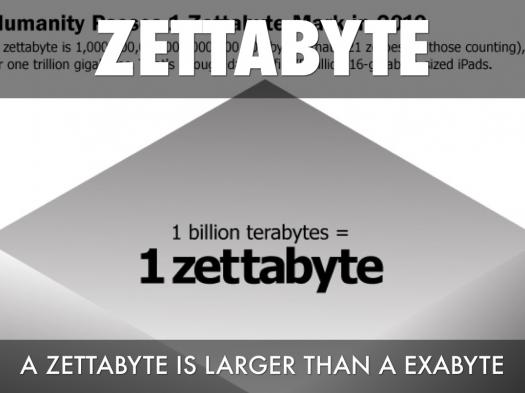# Do You Know A Zettabyte?

Approved & Edited by ProProfs Editorial Team
At ProProfs Quizzes, our dedicated in-house team of experts takes pride in their work. With a sharp eye for detail, they meticulously review each quiz. This ensures that every quiz, taken by over 100 million users, meets our standards of accuracy, clarity, and engagement.
| Written by Linda
L
Linda
Community Contributor
Quizzes Created: 19 | Total Attempts: 1,099
Questions: 10 | Attempts: 27SettingsI must warn you, this quiz is loaded and it might get confusing. Hope you remember your elementary mathematics. That, with a little bit of wit, and you will come out on top.

• 1.

### How many bytes make one zettabyte?

• A.

One zillion

• B.

Two million megabytes

• C.

One sextillion

• D.

One billion

C. One sextillion
Explanation
One zettabyte is equal to one sextillion bytes. The prefix "zetta-" represents a factor of 10^21, which means that there are 1,000,000,000,000,000,000,000 bytes in one zettabyte. Therefore, the correct answer is "One sextillion."

Rate this question:

• 2.

### What is the unit symbol of the zettabyte?

• A.

ZB

• B.

Zb

• C.

Z

• D.

Zb

A. ZB
Explanation
The unit symbol of the zettabyte is ZB. ZB stands for zettabyte and is used to represent a unit of digital information storage. It is equal to 1,000,000,000,000,000,000,000 bytes.

Rate this question:

• 3.

### How many exabytes make up one zettabyte?

• A.

1 000 000

• B.

10 000

• C.

1 000

• D.

100

C. 1 000
Explanation
One zettabyte is equal to 1,000 exabytes. The prefix "zetta" represents a factor of 10^21, while the prefix "exa" represents a factor of 10^18. Therefore, there are 1,000 exabytes in one zettabyte.

Rate this question:

• 4.

### One zettabyte is approximately equal to a billion?

• A.

Megabytes

• B.

Terabytes

• C.

Exabytes

• D.

Nanobytes

B. Terabytes
Explanation
One zettabyte is approximately equal to a billion terabytes. A zettabyte is a unit of digital information that represents 1 trillion gigabytes or 1 billion terabytes. Therefore, the correct answer is terabytes.

Rate this question:

• 5.

### Which of the following best describes a zettabyte?

• A.

10^8

• B.

10^56

• C.

10^21

• D.

10^18

C. 10^21
Explanation
A zettabyte is a unit of digital information storage and it represents 10^21 bytes. It is a very large amount of data, equivalent to one sextillion bytes. This is often used to measure the capacity of storage devices or the amount of data transmitted over a network.

Rate this question:

• 6.

### Which of the following best represents a zettabyte?

• A.

ZY

• B.

ZT

• C.

ZE

• D.

ZB

D. ZB
Explanation
A zettabyte is represented by the abbreviation ZB. The prefix "zetta" denotes a factor of 10^21, which is equivalent to one sextillion bytes. Therefore, ZB is the correct representation for a zettabyte.

Rate this question:

• 7.

### What does zetta in “Zettabyte” mean?

• A.

Twenty

• B.

Seven

• C.

Five

• D.

Fifteen

B. Seven
Explanation
The correct answer is "Seven" because the prefix "zetta" in "Zettabyte" represents a factor of 10^21. In the International System of Units (SI), each prefix denotes a power of 10. Therefore, "zetta" corresponds to 10^21, making it the correct option.

Rate this question:

• 8.

### How many bytes is a zettabyte?

• A.

Million

• B.

Sextillion

• C.

• D.

Trillion

B. Sextillion
Explanation
A zettabyte is a unit of digital information that is equal to 1 sextillion bytes. A sextillion is a number represented by 1 followed by 21 zeros. Therefore, a zettabyte consists of an incredibly large amount of data, making sextillion the correct answer.

Rate this question:

• 9.

### What is the maximum storage of ZFS?

• A.

• B.

• C.

• D.

Explanation
ZFS, or Zettabyte File System, is a high-performance file system that provides advanced data management and protection features. It is designed to handle massive amounts of data, making it suitable for enterprise-level storage solutions. The maximum storage of ZFS is 256 quadrillion, which is an extremely large capacity and allows for the efficient management of vast amounts of data.

Rate this question:

• 10.

### How would you describe a ZB?

• A.

1.181 ZB

• B.

1.57 ZB

• C.

1.23 ZB

• D.

2 ZBBack to top